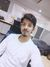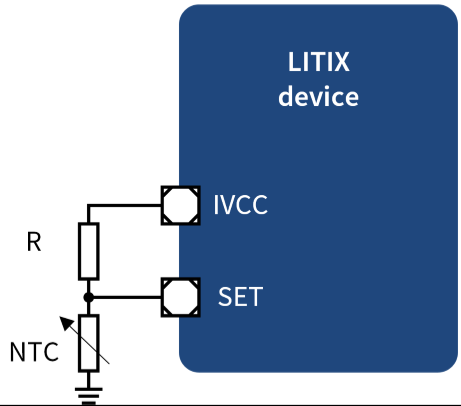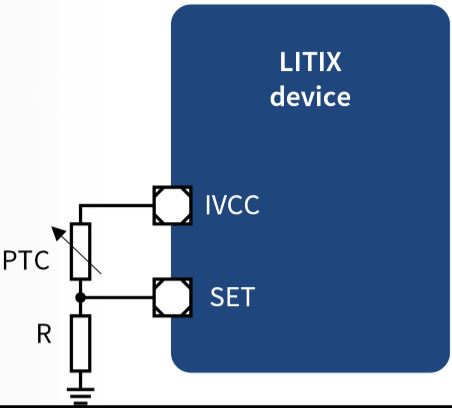# How to protect the LED from overheating?Community ManagerCommunity Manager

# How to protect the LED from overheating?

LEDs transform only 2/3 of the electrical power to light, while 1/3 are dissipated in heat. When the LED junction temperature reaches the maximum rating the system is no longer reliable. In this case an output power reduction strategy allows to keep the LED turned on while not violating the temperature rating.

To apply an output power derating strategy it is required to estimate the junction temperature of LEDs. To do this, a temperature sensing element is placed on the same PCB close to the LEDs. The sensing position determinates the detected temperature as a function of LED junction temperature. Through thermal simulation or experimentally it is possible to relate the sensed temperature  to the actual junction temperature of the LED.

To keep the overall cost of the system low, the most common sensing elements are NTC or PTC resistors. NTCs and  PTCs can be connected to the analog dimming input of LITIX Power devices (SET pin).

NTC resistors reduce their resistance when the sensed  temperature increases. This characteristic can be used to reduce the voltage on SET pin during the overheating by placing the resistor as illustrated belowHints to design a power derating with NTC:

1. Define at which temperature T~a~ the system need to degrade the output power
2. Select an NTC with appropriate R/T characteristic and calculate the resistance at temperature T~a~
3. Calculate R that imposes 1.6 V to SET pin at T~a~ temperature by R{~}NTC~ T~a~)*(5-1.6)/1.6
4. Calculate the overall IVCC current consumption with lowest value of NTC (maximum temperature) and check that it is lower than the maximum allowable.

PTC resistors increase their resistance when the sensed temperature increases. This characteristic can be used to reduce the current on analog dimming network and then the voltage on SET pin. The circuit proposed is the followingHints to design a power derating with PTC:

1. Define at which temperature T~a~ the system need to degrade the output power
2. Select an PTC with appropriate R/T characteristic curve and calculate the resistance at temperature T~a~
3. Calculate R that imposes 1.6 V to SET pin at T~a~ temperature by R{~}PTC~ (T~a~)*1.6/(5-1.6)
4. Calculate the overall IVCC current consumption with lowest value of PTC (minimum temperature) and check that is lower than the maximum allowable.

See https://www.infineon.com/cms/en/product/power/lighting-ics/litix-automotive-led-driver-ic/litix-powe... for datasheets and application notes.

NTC resistors reduce their resistance when the sensed  temperature increases. This characteristic can be used to reduce the voltage on SET pin during the overheating by placing the resistor as illustrated below

Contributors